# Choose the Symbol that Follows the Rule

In this worksheet, students will match pairs of shape-based symbols in the same way as a pair of examples. It will develop their ability to categorise and use skills such as rotation and mirror imaging.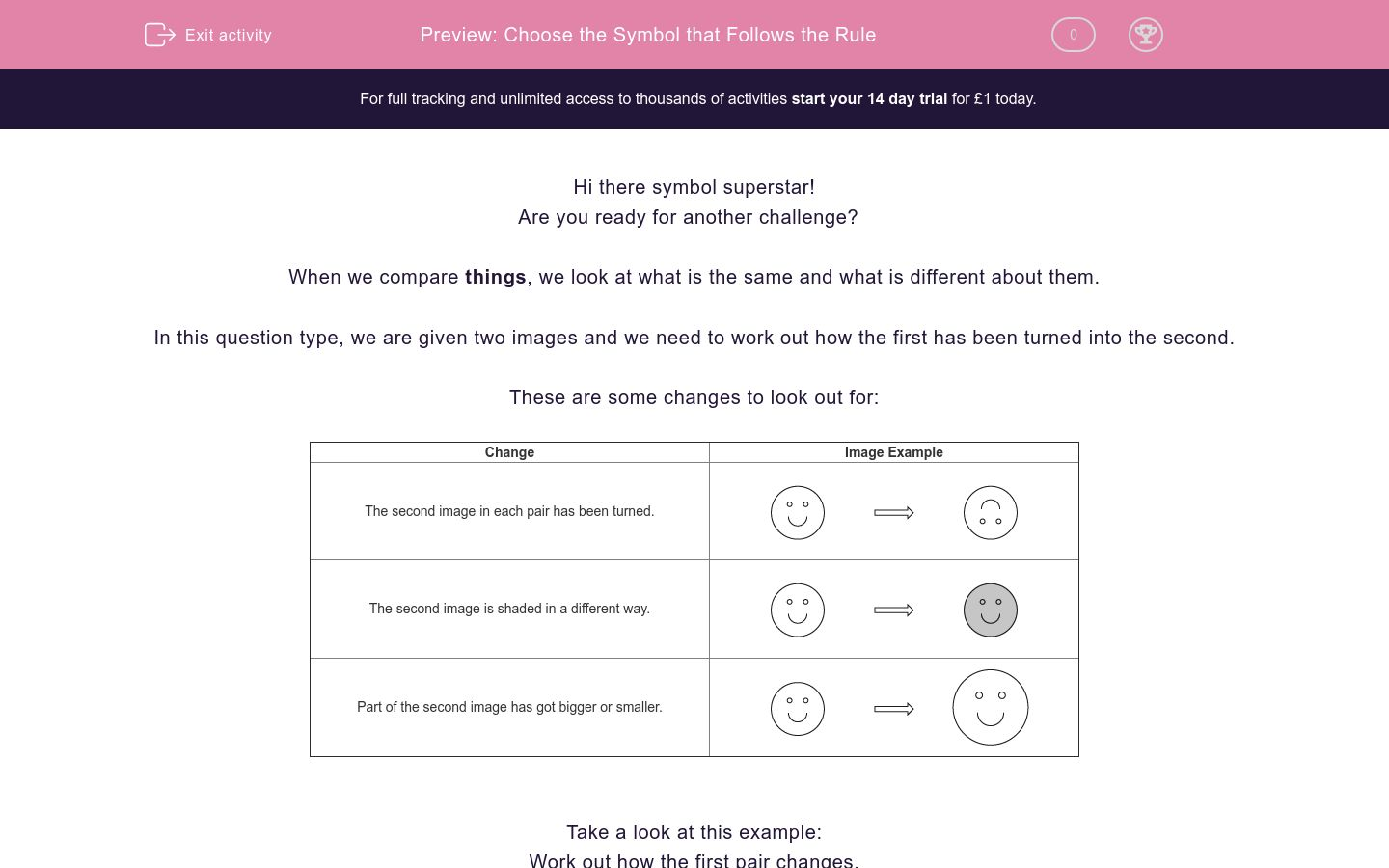Key stage:  KS 2

Curriculum topic:   Spatial and Non-Verbal Reasoning

Curriculum subtopic:   Apply the Relationship

Difficulty level:### QUESTION 1 of 10

Hi there symbol superstar!

Are you ready for another challenge?

When we compare things, we look at what is the same and what is different about them.

In this question type, we are given two images and we need to work out how the first has been turned into the second.

These are some changes to look out for:

 Change Image Example The second image in each pair has been turned.The second image is shaded in a different way.Part of the second image has got bigger or smaller.Take a look at this example:

Work out how the first pair changes.

Then use the same rule to work out which image best completes the second pair.Which of these options would the square turn into?

a)b)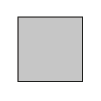c)Did you notice that the shading is darker between the first and second images in the first pair?

Therefore, we must also make the shading darker in the second pair, so the answer which best fits the sequence is b.

In this activity, you will need to be a symbol superstar and match images based on a pattern that you will find.

Remember to work out how the first image changes into the second image to find the rule.

Let’s get started!

Look at the first pair of images here.

Can you see how they are related?

Apply this relationship to the second set by choosing the image from the options below which would best complete the pair.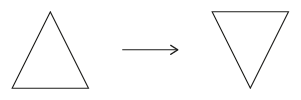Look at the first pair of images here.

Can you see how they are related?

Which image would complete the second set?Look at the first pair of images here.

Can you see how they are related?

Which image would complete the second set?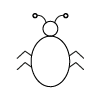Look at the first pair of images here.

Can you see how they are related?

Which image would complete the second set?Look at the first pair of images here.

Can you see how they are related?

Which image would complete the second set?Look at the first pair of images here.

Can you see how they are related?

Which image would complete the second set?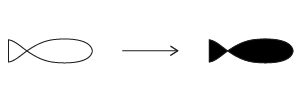Look at the first pair of images here.

Can you see how they are related?

Which image would complete the second set?Look at the first pair of images here.

Can you see how they are related?

Which image would complete the second set?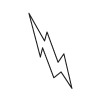Look at the first pair of images here.

Can you see how they are related?

Which image would complete the second set?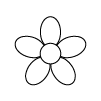Look at the first pair of images here.

Can you see how they are related?

Which image would complete the second set?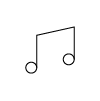• Question 1

Look at the first pair of images here.

Can you see how they are related?

Apply this relationship to the second set by choosing the image from the options below which would best complete the pair.EDDIE SAYS
The first triangle has been mirrored to become the second triangle in the pair. This means that we need to mirror the second triangle in the same way. So option b is the correct answer. Try not to get caught out by option c as the shape is the wrong colour. We need to look out for red herrings like this which aim to throw us off the right path as a detective!
• Question 2

Look at the first pair of images here.

Can you see how they are related?

Which image would complete the second set?EDDIE SAYS
How did you do symbol detective? The first flower becomes black in the second image. If we apply this rule to the heart, we need to find the option that represents a black heart. This means that option a is the correct answer.
• Question 3

Look at the first pair of images here.

Can you see how they are related?

Which image would complete the second set?EDDIE SAYS
Did you spot that the smiley face got bigger? This means that we need to make the bug bigger too. The colour of the bug does not need to change though. This means that option c is the image that would best complete the second pair. I'm itching at the sight of that big bug!
• Question 4

Look at the first pair of images here.

Can you see how they are related?

Which image would complete the second set?EDDIE SAYS
Nice try detective! In this question, you need to spot the differences in the shading. In the first pair, the square has turned from black to white. If we do the same to the first image in the second pair, we know that we are looking for a white triangle. This makes option b the correct answer.
• Question 5

Look at the first pair of images here.

Can you see how they are related?

Which image would complete the second set?EDDIE SAYS
In the first pair, the circle is getting much smaller. So we need to make the heart much smaller in the same way. There is no need to change the colour of the heart, as no colour change occurs in the first pair. This makes option a the best fit in the second pair. I hope you are LOVING these questions so far!
• Question 6

Look at the first pair of images here.

Can you see how they are related?

Which image would complete the second set?EDDIE SAYS
Did you work this one out detective? This is another question which focuses on shading. The fish in the first pair changes from white to black. If we apply this change to the second pair, we know we are looking for a black circle. This makes option b the correct answer. Don't be fooled by option c as the circle is too big!
• Question 7

Look at the first pair of images here.

Can you see how they are related?

Which image would complete the second set?EDDIE SAYS
Your rotation skills are important in this question detective. The first heart has been flipped upside down or rotated through a half turn (or 180 degrees). So we need to flip the water droplet upside down in the same way, which makes option a the correct answer.
• Question 8

Look at the first pair of images here.

Can you see how they are related?

Which image would complete the second set?EDDIE SAYS
The boats in the first pair has progressed in colour from grey to black. We need to apply the same change to the lightning bolt. This makes option c the correct answer in this pair. Let's take a look at the next one detective.
• Question 9

Look at the first pair of images here.

Can you see how they are related?

Which image would complete the second set?EDDIE SAYS
Did your detective's eyes spot that the images are getting bigger here? I'd love to be able to make my cakes bigger in reality - YUM! We need to find a bigger version of the same flower to fit in the second pair. This makes option c the correct answer.
• Question 10

Look at the first pair of images here.

Can you see how they are related?

Which image would complete the second set?EDDIE SAYS
The last one is another shading question. The images are moving from white to black, so we need to find a black musical note to complete the pattern. This makes option b the correct fit. Well done for applying all your observations in this activity top detective! Remember to always look for changes in size, position or shading as your first clue to the correct answer.
---- OR ----

Sign up for a £1 trial so you can track and measure your child's progress on this activity.

### What is EdPlace?

We're your National Curriculum aligned online education content provider helping each child succeed in English, maths and science from year 1 to GCSE. With an EdPlace account you’ll be able to track and measure progress, helping each child achieve their best. We build confidence and attainment by personalising each child’s learning at a level that suits them.

Get started